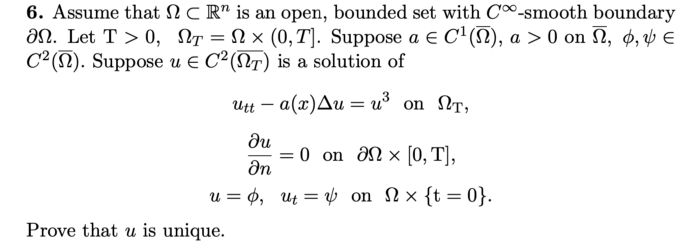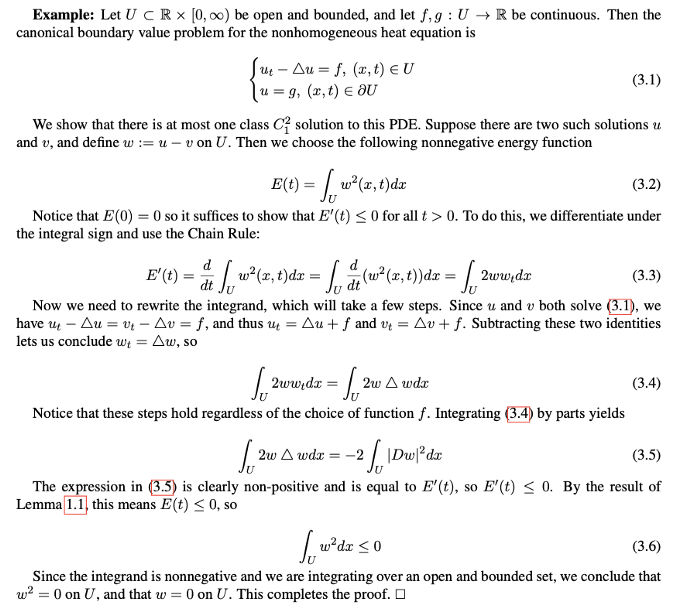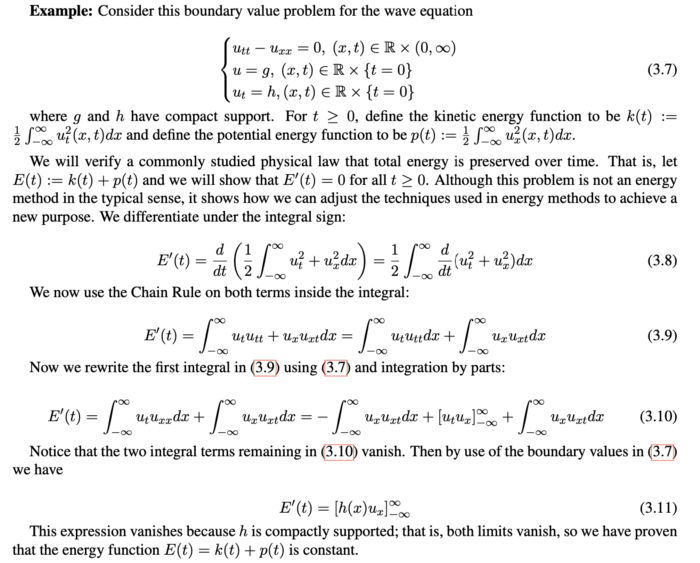# Before we get into the details of the energy method, we need one other tool that fuels the fire: a derivative comparison.Joshua Siktar

2 years ago | 6 min read

An ordinary differential equation (ODE) is an equation involving a scalar-valued function from the real line to the real line, and some of its derivatives. A partial differential equation (PDE) is a generalization of the ordinary differential equation to the event where the argument is in multiple dimensions. In this case we have partial derivatives in each direction.

For example, if the input is an ordered pair, then the input belongs to R², so we may have partial derivatives in two different directions. No matter if we’re dealing with an ODE or a PDE, a solution will be a function that you can plug into the equation and satisfy it.

For any given ODE or PDE, there might be one solution; there might be multiple solutions; or there might not be any at all. The purpose of an energy method is to help answer this question. Here is an [admittedly very difficult] energy method problem from the qualifying exam I took at the University of Tennessee-Knoxville.A problem from the August 2020 University of Tennessee PDE prelim. This can be solved using the infamous energy method.

Even if you don’t understand all the terminology and notation in the problem, the goal of an energy method should remain clear: investigate whether there can be multiple solutions to an ODE (or PDE), or not. Sometimes this is paired with finding an explicit solution, because of the following key idea:

If there is at least one solution, and there is at most one solution, then the solution is unique.

This statement may seem obvious but it is very powerful, because many methods for finding exact solutions do not have a built-in tool to check if they are the only solutions.

There are lots of techniques for finding exact solutions to ODEs and PDEs, including: the Laplace Transform, the Fourier transform, separation of variables, and Duhamel’s Formula.

My focus will not be on these techniques, but rather on the more abstract way of determining whether such solutions are the only ones. So, once you have a solution, the energy method is one way to determine it is the only one. This is very exciting because one cannot possibly hope to check, by hand, that every other possible function is not a solution.

Before we get into the details of the energy method, we need one other tool that fuels the fire: a derivative comparison.

Derivative Comparisons

What is a derivative comparison? This general term can refer to lots of different statements, but the one of interest right now is below:

The ultimate goal is comparing two differentiable functions where a relationship between their derivatives is known. To use this tool, of course we have to know before hand that the two functions in question are once-differentiable (i.e. have one derivative).

In words, it says that if two functions are differentiable on an interval and one of those functions is “ahead” of the other and continues to outpace it, the other function cannot catch up. This can also be extended to the entire real line if you use some point on the real line as your basis of comparison.

Finally, there is an analogue of this derivative comparison for functions defined on Euclidean space in arbitrarily many dimensions, that uses partial derivatives. I’ll let you, the reader, put that carefully and precisely into words.

Now we can move onto the actual energy methods.

The Idea of an Energy Method

If we want to show that an ODE or PDE has at most one solution, we pretend there are two distinct solutions, and try to prove that this is impossible, therefore reaching a contradiction. Let’s say one of those solutions is called u, and the other is called v.

In particular, we choose E so that E(0) = 0, and then compute E′(t) allowing differentiation under the integral sign or use of the Fundamental Theorem of Calculus if needed. Upon utilizing a carefully selected chain of inequalities we aim to conclude that E′(t) ≤ 0, and hence, by using Lemma 1.1, further conclude that E(t) ≤ 0. Moreover, if the integrand is a priori nonnegative, then it must be zero. This lets us conclude that if a solution u exists, then it is unique.

It is worth noting that the exact procedure used to show uniqueness of solutions will vary slightly, and oftentimes a great deal of trial and error, in particular in choosing the function E, will be necessary.

Moreover, we will not directly discuss existence of solutions in this article, as the concept of an energy method fundamentally rests on assuming a solution exists, even from the first step. Simply knowing there is at most one solution is a lot less valuable than knowing there is exactly one solution.

Example with an ODE

As you might expect, here is a specific example of showing how the energy method works for an ordinary differential equation. This particular problem is one of many initial-value problems, where an ODE is paired with exact knowledge of how the function behaves at time t = 0.

Energy Methods for PDEs

Even though energy methods are conceptually more straightforward with ordinary differential equations, they were traditionally introduced for use in partial differential equations.

Unlike for energy methods for ODEs, the energy functions for PDEs will generally be integral expressions. This allows us to utilize properties of integrals of signed functions.Uniqueness of solution to an PDE with energy method (heat eqauation boundary value problem)

As a side note, this problem is a boundary-value problem since information of a solution on the boundary of the domain is given. There are two recurring patterns that this example helps to illustrate.

The first of these patterns is the idea used at the beginning of the proof: assume [for sake of contradiction] that there are two distinct solutions to the PDE (or ODE), and use some other variable to denote the difference of these two solutions; the proof will be complete when it is shown that this difference equals zero on the entire domain of the PDE (or ODE).

The second recurring theme appears at the end of this proof: we have found a nonnegative integrand, integrated it, and shown that the integrated expression is non-positive. Since the integrand itself is non-negative, it must, automatically, equal zero.

There is a technicality here pertaining to sets of Lebesgue measure zero — that may sound familiar to you if you’ve studied measure theory — but assuming continuity of the solutions circumvents that entirely. It is fine to skip this technicality as long as we are dealing with strong, or classical, solutions to an ODE or PDE.

More advanced problems not covered here may instead deal with weak solutions to PDEs on Sobolev Spaces, where continuity is no longer guaranteed (or even necessary).

Some Other Explorations: Conservation of Energy

The next example has a slightly different goal that among other things, allows us to see how the energy method got its name. This problem will take an energy function — an integral expression — and show that it is constant over time, or that its derivative with respect to time is equal to zero. Such a problem is called a conservation of energy.Conservation of the energy functional for the wave equation initial value problem (IVP)

Notice that unlike the heat equation problem, here we are given information about the derivative of u at time t = 0. This is characteristic of hyperbolic PDE problems, including the wave equation.

One other problem to try

Here is one other problem you could consider tackling if you want extra practice, particularly the first part.

This is another hyperbolic problem. While maximum principles can often be used in lieu of energy methods to prove the uniqueness of solution to a PDE, the maximum principle doesn’t generally work for hyperbolic problems such as this one, due to the lack of a first-order time derivative appearing in the PDE itself. In other words, try an energy method, using the techniques we talked about in this article.

Many graduate-level PDE textbooks — namely the one by Evans — will provide plenty of other examples of energy method problems for elliptic, parabolic, and hyperbolic PDEs. Specific examples include the Poisson Equation, the Laplace Equation, the heat equation, and both linear and nonlinear variants of the wave equation.

UpvoteCreated by

Joshua Siktar

Ph.D. Candidate, Applied Mathematics, University of Tennessee-Knoxville | B.S. Mathematics, Carnegie Mellon | Facilitator of Modernization of EducationPost

Upvote

Downvote

Comment

Bookmark

Share

Related Articles